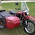## 11 January 2015

### Problem:

Given distances between different vertices, find the shortest path from vertex i to vertex j

### Input Format:

N ie no of direct path from one vertex to another
Next N lines have format- vertexA vertexB distance
vertexI vertexJ

### Output Format:

shortest path
length of shortest path

Constraints:
None

11
a b 5
c a 5
a d 10
e c 12
d f 13
f d 1
a e 2
e a 4
f e 2
e f 1
b d 3
a d

a e f d
4

### Explanations:

shortest path from a to d is a-e-f-d

Solution:

#!/usr/bin/perl
use warnings;
use strict;

use Graph;
use Graph::Directed;

my \$g = 'Graph::Directed'->new;

chomp(my \$n=<>);
for(my \$i=0;\$i<\$n;\$i++)
{
my \$tmp=<>;
my @arr=split(" ",\$tmp);
\$g->add_weighted_edges(@arr);
}

my \$APSP = \$g->APSP_Floyd_Warshall;

chomp(my \$in=<>);
(my \$u,my \$v)=split(" ",\$in);
my \$w = \$APSP->path_length(\$u, \$v);

if (\$w) {
my @p = \$APSP->path_vertices(\$u, \$v);
foreach(@p)
{
print "\$_ ";
}
print "\n\$w";
}

### Tips:

Use module Graph for such problems

#### 2 comments:

1.Your sample input file starts with 11, so the program expects 11 lines of graph definition, but you only supply 10. Your program then dies with "Uninitialized value for \$n" in line 21. You either need to add an additional graph definition line, or change the first line to 10.

2.Added an extra line, copy/paste error. Thanks for pointing.